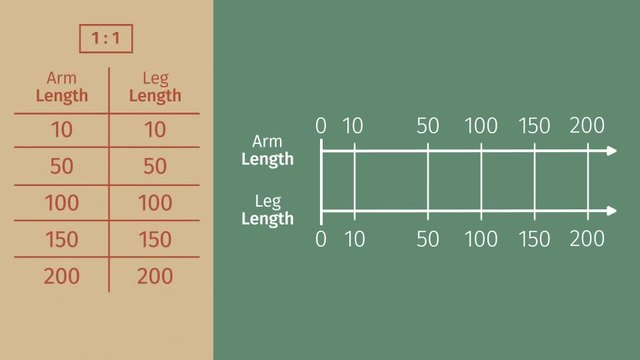# From Ratio Tables to Double Number LinesRating

Be the first to give a rating!

The authorsChris Reger

## Basics on the topicFrom Ratio Tables to Double Number Lines

After this lesson, you will be able to transform ratio tables into double number line diagrams.

The lesson begins by teaching you that equivalent ratios can be presented in a ratio table. It leads you to learn how to rewrite the entries in a ratio table horizontally to produce a double number line diagram. It concludes with a hint that double number line diagrams are more visually-friendly when comparing ratios.

Learn about transforming ratio tables into double number line diagrams by helping Mary build a monster!

This video includes key concepts, notation, and vocabulary such as ratio (a relationship between two non-negative numbers, both of which are not zero); ratio table (a structured set of equivalent ratios); equivalent ratios (ratios showing the same relationship when expressed in the simplest form); and double number line diagram (a diagram using 2 horizontal lines and ticks to show a set of equivalent ratios).

Before watching this video, you should already be familiar with ratio table and equivalent ratios.

After watching this video, you will be prepared to learn how to solve real-world and mathematical problems involving equivalent ratios, unit rate, percent, and measurement conversion.

Common Core Standard(s) in focus: 6.RP.A.3 A video intended for math students in the 6th grade Recommended for students who are 11 - 12 years old